## General Chemistry

During the electrolysis of water, hydrogen and oxygen gases are produced according to the following chemical equation:

2H2O(l) → 2H2(g) + O2(g

Notice that free elements are formed from a compound which indicates that this is a redox reaction where hydrogen is reduced, and the oxygen is oxidized: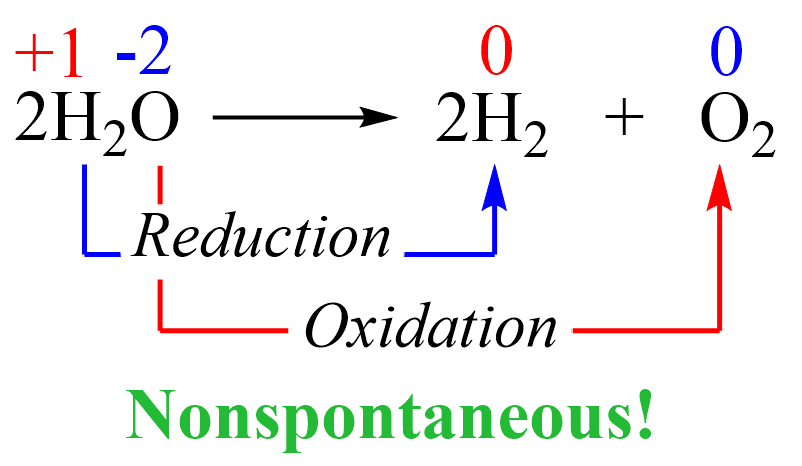This reaction is not spontaneous as we all know that water does not convert into hydrogen and oxygen. On the contrary, the reverse reaction is not only spontaneous but also explosive since hydrogen is burned in oxygen vigorously: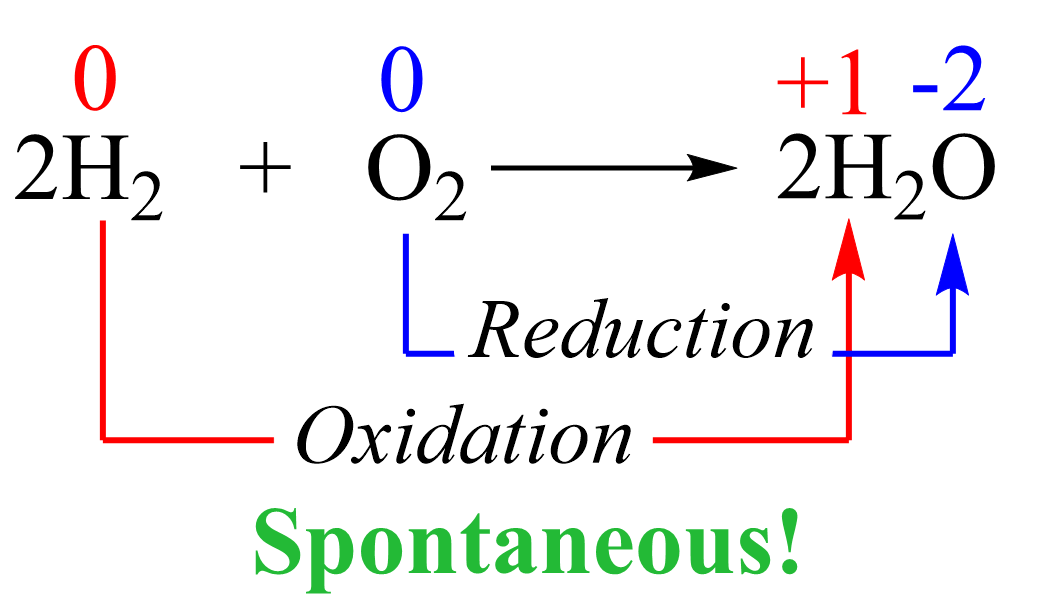Therefore, in order to break down the water into elements, we need external energy input. Remember, from thermodynamics that if a process is endothermic (more accurately endergonic if we are talking of the Gibbs free energy), then we need to supply the necessary amount of energy to push it forward. The energy for the decomposition of water is +474.4 kJ/mol and this would be the minimum energy needed to accomplish this transformation: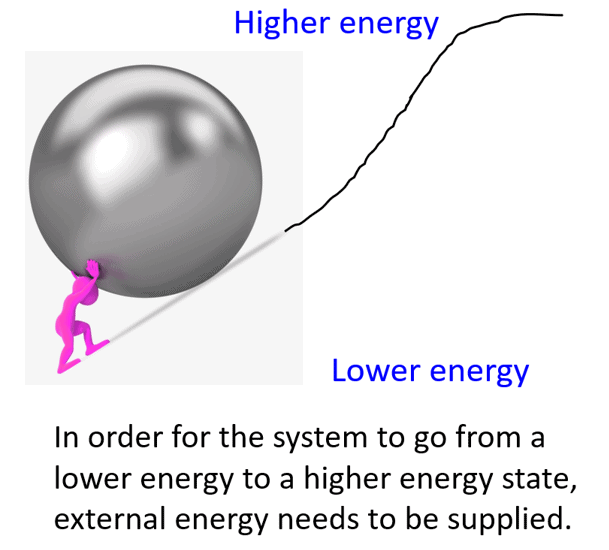In the electrolysis of water, or any other species, this energy is provided via external electrical power, and the reaction is run in an electrolytic cell. Electrolysis is a reaction where electrical current drives an otherwise nonspontaneous redox reaction through a process. We have seen how it works for molten and aqueous NaCl, so feel free to check this article for more details before moving forward.

# Electrolysis of Water

The first thing we will need to do electrolysis of water is a battery with an estimated potential of greater than 1.23 V as the estimated cell potential is -1.23 V (see later). We are also going to need two electrodes (anode and cathode) which, unlike galvanic cells, are typically made of the same metal as it is not part of the reaction. Now, just inserting two powered electrodes in pure water will not cause electrolysis because to have a current flow, we need some electrolyte. This can be any ionic compound with good solubility that is not at risk of undergoing oxidation or reduction at the given condition. For example, we have seen earlier that NaCl may undergo electrolysis in an aqueous solution, but let’s say Na2SO4 does not pose that risk. So, let’s draw what we have so far for the electrolytic cell: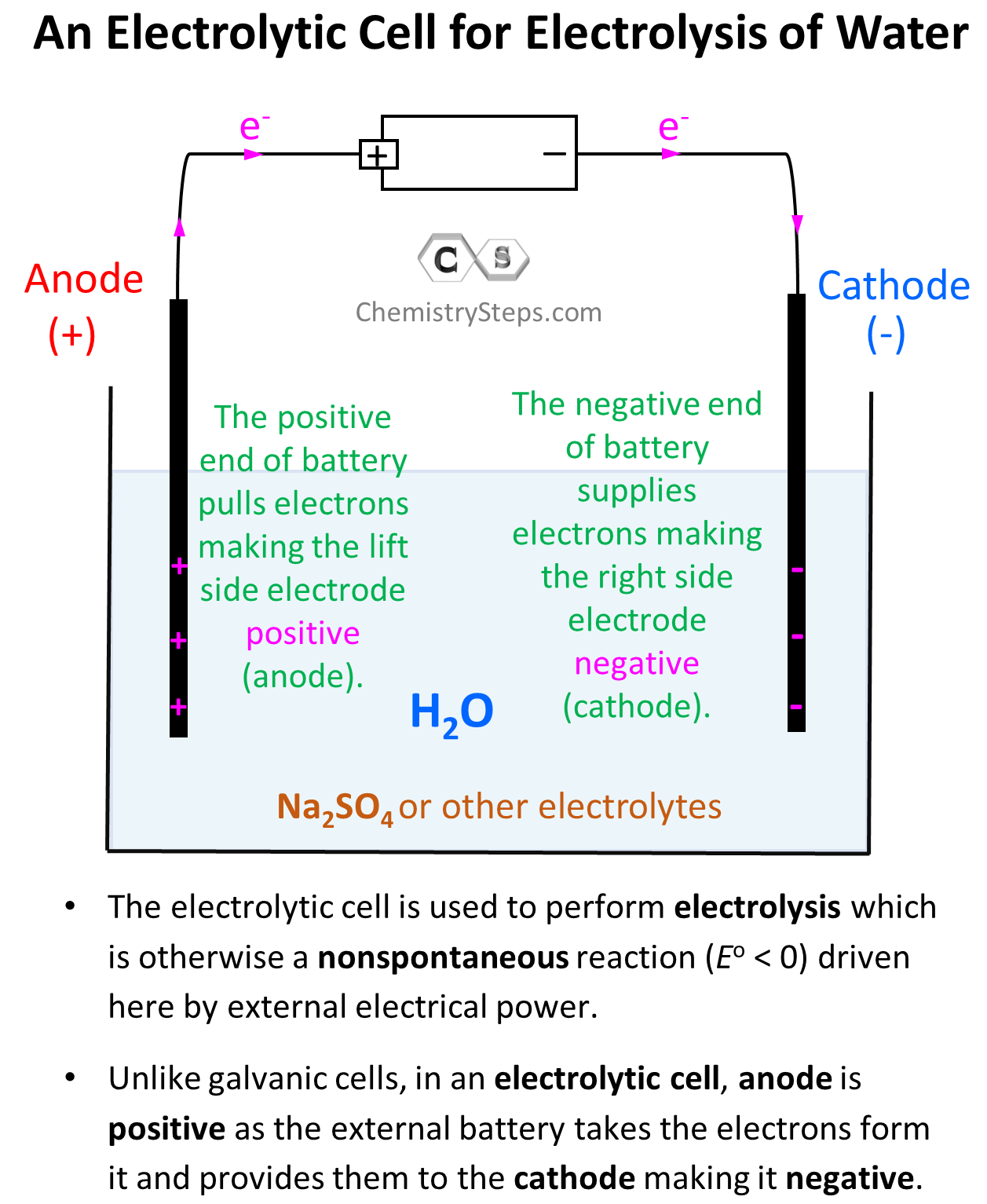The electrode on the left side is connected to the positive end of the battery and therefore, it is positively charged, and the one on the right side is negatively charged.

So, what happens with the water molecules in the presence of these electrodes?

Let’s first recall that water is a polar compound which is why it is actually such a good solvent for all the salts and minerals that we know. Remember, because of greater electronegativity, the oxygen is partially negatively charged, and the two hydrogens are partially positively charged: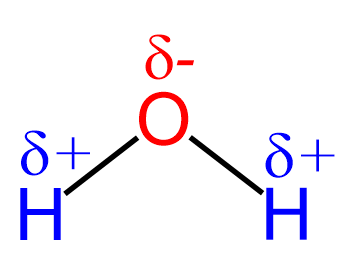Oxygen has greater electronegativity, so it pulls one electron from each bond. Therefore, it is partially negatively charged, and the oxidation number is -2. Hydrogen has lower electronegativity, and it gives the electron to oxygen, thus is partially positively charged with an oxidation number of +1.

We can visualize the beginning of the electrolysis as the negative end of water molecules surrounding the anode (+ in the case of electrolytic cells), and the positive end of the molecule surrounding the cathode (- in electrolytic cells):After this, the negatively charged electrode is going to push electrons to the electron-deficient H atoms (Hδ+) thus reducing them (cathode) to H0 – bubbling H2 gas. On the other hand, the positively charged electrode needs electrons it is going to pull them from the Oδ– atoms oxidizing them to O2 gas. This is the oxidation half-reaction and therefore, the electrode is the anode: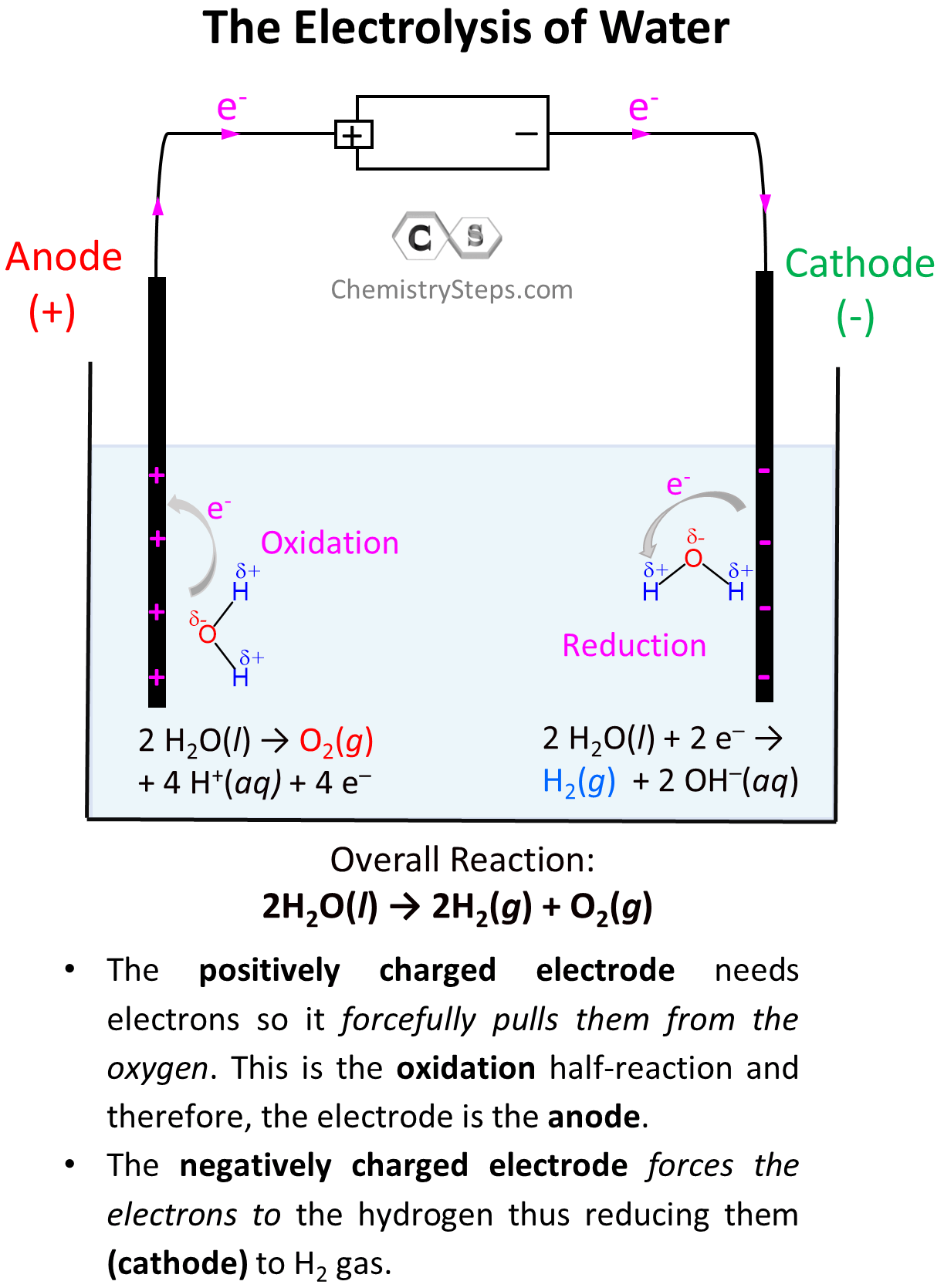So, on the left electrode, oxygen atoms are being oxidized to O2, and therefore, this is the anode, while the H atoms are being reduced on the right side electrode making it the cathode.

Remember, regardless of the type of electrochemical cell, oxidation always occurs on the anode, and the reduction occurs on the cathode.

Notice, however, that the anode is positively charged here unlike what we saw in galvanic cells, where it was considered to be negatively charged as it gave the electrons to the cathode thus undergoing oxidation. In electrolytic cells, the external power source draws the electrons from the anode thus making it positive. So, keep in mind:

• In a voltaic cell, the anode is negative as it takes the electrons from the cathode and thus making positive.
• In an electrolytic cell, the anode is positive as the external battery takes the electrons from it, and provides them to the cathode making it negative.

Let’s now write down each half-reaction. On the anode, we have the oxidation of water, and on the cathode, we have the reduction of it:

Oxidation (anode): 2 H2O(l) → O2(g) + 4 H+(aq) + 4 e   E° = -1.23 V

Reduction (cathode): 2 H2O(l) + 2 e → H2(g)  + 2 OH(aq)    E° = -0.83 V

The H+ and OH ions combine to form water, so the overall reaction then will be written as:

2H2O(l) → 2H2(g) + O2(gE° = -1.23 V

Note: The cell potentials given above are for standard conditions which imply 1 M H+. However, at pH 7, [H+] = [OH] = 10-7 M, so we do not have standard conditions, and the cell potentials are 0.82 V and -0.41 V respectively.

Notice also that the oxidation half-reaction is reversed compared to how it is shown in the tables of standard reduction potential (O2(g) + 4 H+(aq) + 4 e → 2 H2O(l) E° = +1.23 V), and that is why we also changed the sign to negative. When one of the reactions is reversed and thus the sign of Eo is changed, we can find the cell potential by adding those of anode and cathode, so here, it would be:

Eocell = -1.23 + (-0.83) = – 2.06 V

This is for standard conditions, and for pH = 7, we’d have:

Ecell = -0.82 + (-0.41) = – 1.23 V

There is an article on calculating the cell potential so feel free to check that if the process is not clear.

The cell potential shows that in order to perform electrolysis of water, the external power supply should be greater than 1.23 V.

# Competing Redox Reactions

One thing worth considering is the possible reduction of Na+ ions at the cathode as this is a competing reaction with the reduction of water:

Na+(aq) + e- → Na(s)  E° = -2.71 V

2 H2O(l)  + 2 e → H2(g)  + 2 OH(aq)  E° = -0.83 V

How do we know which reduction will occur at the anode? In general, the species that are easier to be reduced or oxidized are going to undergo the reaction. In this case, the Eo values are quite different, and as expected, because of a higher (less largely negative) standard reduction potential, water is reduced preferentially producing bubbles of hydrogen gas at the cathode. Sometimes, when the E° values are close, the species with more negative reduction potential are reduced because of what is called overpotential. This is observed during the electrolysis of aqueous NaCl, so feel free to check that here as well.

In the end, remember this summary of electrolysis and how it compares to spontaneous redox reactions in galvanic cells:

• Electrolysis is performed in an electrolytic cell, and it is the process of driving a nonspontaneous redox reaction by using external electrical power
• Both in galvanic and electrolytic cells, oxidation always takes place on the anode, and the reduction takes place on the cathode.
• In a voltaic cell, the anode is negative as it takes the electrons from the cathode and thus making positive.
• In an electrolytic cell, the anode is positive as the external battery takes the electrons from it and provides them to the cathode making it negative.

Electrolysis also has numerous other applications. For example, fluorine cannot be prepared by any spontaneous chemical reaction. It was found in 1886 by the French chemist Henri Moissan that passing electrical current through a molten mixture of potassium fluoride and hydrogen fluoride produces Fluorine, and it is still prepared commercially by the same method. Another application of electrolysis is the preparation of metals from their oxides which is how most metals are found in Earth’s crust.